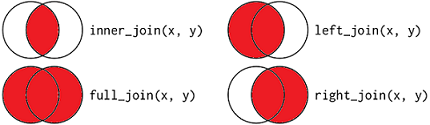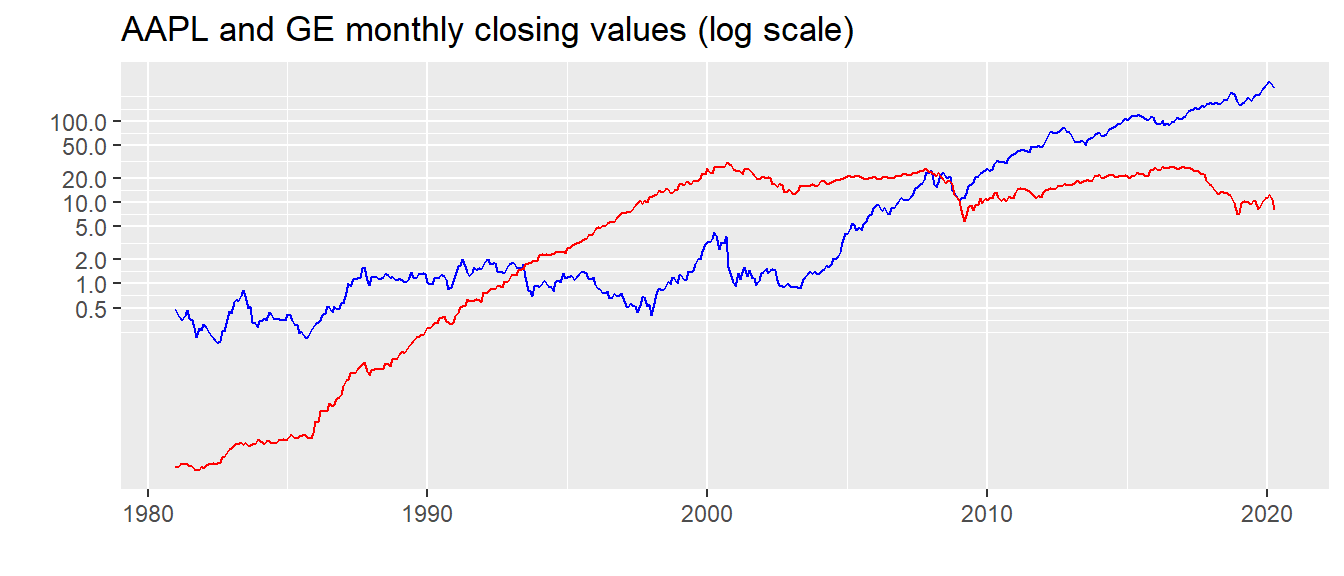# Lab 3: Joining data frames

### Learning objectives:

• the pipe operator %>%
• joining data frames using full_join() and inner_join()
• filtering out rows with missing observations using is.na() function
• creating descriptive statistics table using stargazer()
• selecting columns using select(), subset(), or [,]
• saving data using write_csv()

### 0.Introduction

Let’s begin by reading in our AAPL and GE data.

library(tidyverse)
library(lubridate)
aapl <- read_csv("https://query1.finance.yahoo.com/v7/finance/download/AAPL?period1=1&period2=2000000000&interval=1d&events=history",
col_types=cols(Date=col_date(),
Adj Close=col_double()))
ge <- read_csv("https://query1.finance.yahoo.com/v7/finance/download/GE?period1=1&period2=2000000000&interval=1d&events=history")

### 1. Using the pipe %>% operator

The pipe operator %>% takes output from preceding function and feeds it as input to the next function. For example, df <- df %>% arrange(Date) is equivalent to df <- arrange(df, Date).

This is useful if we want to apply several functions in succession to a data frame. For example, when we load our aapl data we want to arrange the data frame, then filter it, then rename and select some variables. We can do this as follows.

aapl <- aapl %>%
arrange(Date) %>%
filter(month(Date) !=lead(month(Date))) %>%
rename(aapl=Adj Close) %>%
select(Date, aapl)

ge <- ge %>%
arrange(Date) %>%
filter(month(Date) !=lead(month(Date))) %>%
rename(ge=Adj Close) %>%
select(Date, ge)

### 2. Joining data frames

We would like to merge the aapl and ge data frames into one. In this case we want to put them side by side. The two data frames have different number of observations - GE begins in 1962, the AAPL in 1980. When putting the data side by side, we need to make sure that rows are correctly aligned by Date. We will do this using function full_join(). The function takes two data frames as the first two arguments and a “by” variable as the third argument.

merged <- full_join(aapl, ge, by="Date")
merged <- arrange(merged, Date)
head(merged, n=3)
## # A tibble: 3 x 3
##   Date        aapl      ge
##   <date>     <dbl>   <dbl>
## 1 1970-01-30    NA 0.00212
## 2 1970-02-27    NA 0.00206
## 3 1970-03-31    NA 0.00217

How many observations should the merged data frame have? full_join() matches the rows that have the same date in each data frame. If dates are found in ge but not in aapl or vice versa, full_join keeps those observations in the merged data frame. It places NAs where it can’t find matches. Since all dates in aapl are also in ge, but not all dates in ge are in aapl, the number of observations should be equal to the number of observations in ge.

There are several different types of joins. They are summarized in the diagram below.For example, if we wanted only observations that are in both aapl and ge, we could use inner_join().

merged <- inner_join(ge, aapl, by="Date")
merged <- arrange(merged, Date)
head(merged, n=3)
## # A tibble: 3 x 3
##   Date            ge  aapl
##   <date>       <dbl> <dbl>
## 1 1980-12-31 0.00540 0.483
## 2 1981-01-30 0.00540 0.400
## 3 1981-02-27 0.00589 0.375

Quick check: Which observations would we get if we ran merged <- left_join(ge, aapl, by="Date")?

What about merged <- left_join(aapl, ge, by="Date")?

Now that we have both GE and AAPL in one data frame we can plot them together.

ggplot(merged, aes(x=Date)) +
geom_line(aes(y=aapl), color="blue") +
geom_line(aes(y=ge), color="red") +
scale_y_continuous(trans="log", breaks=c(0.5,1,2,5,10,20,50,100)) +
labs(title="AAPL and GE monthly closing values (log scale)") + ylab("") + xlab("")### 3. Filtering out rows with missing observations using is.na() function

Suppose that instead of using inner_join() we had used full_join() and wanted to filter out dates that have missing observations for aapl. We can use function is.na(). This function returns value TRUE if the expression inside it is NA, and FALSE if the expression is not NA. We only want non-missing values so we keep values when is.na(NASDQ) is FALSE. Note the == sign indicates equal, != indicates not equal, | indicates or.

merged <- full_join(aapl, ge, by="Date")
merged <- filter(merged, is.na(aapl) == FALSE)

We would have accomplished the same thing using merged <- filter(merged, !is.na(aapl)). The exclamation mark “!” stands for logical expression ‘not’, so !is.na(NASDQ) is TRUE when aapl is not missing.

### IN-CLASS EXERCISE

1. Load in the NHL data on games and results, and NHL data on arenas. What is the number of observations in each data frame?
nhl <- read_csv("https://dvorakt.github.io/business_analytics/lab1/NHLseason2016.csv")
arenas <- read_csv("https://dvorakt.github.io/business_analytics/lab3/NHLarenas.csv")
1. Are all teams in arenas also in nhl? Are all teams in nhl also in arenas?

2. What would be the best way to join the two data frames? Which variable should be the “by” variable?

3. Calculate percent occupancy for each game (i.e. attendance/capacity).

### 4. Creating a descriptive statistics table

Let’s calculate create a table with summary statistics of monthly returns on S&P and NASDAQ.

merged <- merged %>%
mutate(ge_ret = (ge - lag(ge))/lag(ge)*100,
aapl_ret = (aapl - lag(aapl))/lag(aapl)*100)

We can either use the base R function summary() or function stargazer() from a package of the same name. The stargazer() produces tables that are somewhat more readable. You can read about stargazer’s capabilities here. Stargazer works best with data frames that are explicitly declared as data frames, so we declare our tibble a data frame.

library(stargazer)
merged <- as.data.frame(merged)
stargazer(merged, type="text", median=TRUE) 
##
## ======================================================================
## Statistic  N   Mean  St. Dev.   Min   Pctl(25) Median Pctl(75)   Max
## ----------------------------------------------------------------------
## aapl      472 30.744  56.879   0.180   0.912   1.465   31.357  308.777
## ge        472 10.806  9.283    0.005   0.376   11.185  19.479  30.299
## ge_ret    471 1.913   8.499   -27.697  -2.555  0.971   6.107   42.119
## aapl_ret  471 2.213   13.100  -57.744  -5.759  2.028   9.844   45.378
## ----------------------------------------------------------------------

### 5. Selecting columns using select(), subset(), or [,]

In the above table, we are not interested in the descriptive statistics of the level of stock prices. We should tell stargazer to use only the two columns with returns. We can do that in a number of ways: using function select(), using base function subset(), or using [,] which allow us to identify which rows and columns we want to use. Notice we nest these functions inside the stargazer() function.

stargazer(select(merged, ge_ret,aapl_ret), type="text", median=TRUE)
##
## ====================================================================
## Statistic  N  Mean  St. Dev.   Min   Pctl(25) Median Pctl(75)  Max
## --------------------------------------------------------------------
## ge_ret    471 1.913  8.499   -27.697  -2.555  0.971   6.107   42.119
## aapl_ret  471 2.213  13.100  -57.744  -5.759  2.028   9.844   45.378
## --------------------------------------------------------------------
stargazer(subset(merged, select=c("ge_ret","aapl_ret")), type="text", median=TRUE) 
##
## ====================================================================
## Statistic  N  Mean  St. Dev.   Min   Pctl(25) Median Pctl(75)  Max
## --------------------------------------------------------------------
## ge_ret    471 1.913  8.499   -27.697  -2.555  0.971   6.107   42.119
## aapl_ret  471 2.213  13.100  -57.744  -5.759  2.028   9.844   45.378
## --------------------------------------------------------------------
stargazer(merged[,c("ge_ret","aapl_ret")], type="text", median=TRUE) 
##
## ====================================================================
## Statistic  N  Mean  St. Dev.   Min   Pctl(25) Median Pctl(75)  Max
## --------------------------------------------------------------------
## ge_ret    471 1.913  8.499   -27.697  -2.555  0.971   6.107   42.119
## aapl_ret  471 2.213  13.100  -57.744  -5.759  2.028   9.844   45.378
## --------------------------------------------------------------------

Let’s also calculate the correlation coefficient between the returns on the two stocks.

cor(merged$ge_ret, merged$aapl_ret, use = "complete.obs")
##  0.2062468

### 6. Saving data using write_csv()

We did some data manipulation and created new data sets that we may want to use in the next lab. Let’s save the data so that we don’t have to redo all of that manipulation. We’ll use function write_csv() which takes as arguments the data frame to be saved and the name of the new .csv file. If you don’t include a full path, the file will be saved in your working directory. To see what is your working directory type getwd() in the console. If you are working within an R Studio project, the working directory is the project’ directory.

write_csv(merged, "GE_AAPL_monthly.csv")
write_csv(nhl, "NHLseason_with_arenas.csv")

### Exercises

1. Data on New York City weather in Nexus lab data files folder in NYC_weather.csv. Save the file to your business analytics folder. The data has information on daily precipitation (in tenths of millimeters) and min and max temperatures (in tenths of degree Celsius) as recorded by the Belevedere Tower in Central Park. The data comes from http://www.ncdc.noaa.gov. Note that missing values are indicated as -9999. Load the data into R.

2. Summarize the variables. Are the values reasonable? Are there any missing values? If so, drop the observations that have missing values. How many observations did you lose by dropping these?

3. Change the DATE variable from a ‘numeric’ type to ‘Date’ type. (Hint 1: as.Date() does not work on numeric, so you first need to turn DATE into character using as.character() Hint 2: Note also that the format of the date is “%Y%m%d” i.e. no dashes between year, month and day.)

4. Plot the minimum and maximum temperatures on a time line for the entire period. Then do the same since 2014.

5. Load in the daily data on Dow Jones Industrial from Nexus. The file is called dji.csv. Calculate daily returns. Check the descriptive stats of your daily return.

6. Merge your daily DJI data with your NYC weather data. Keep only dates that are in both data sets. Which observations do you lose from either data set?

7. What is the correlation between temperature and returns?

8. Save the merged data into a .csv file. Make sure that you are in your business analytics project` so that R knows to save into that folder.